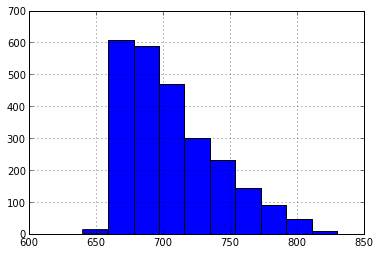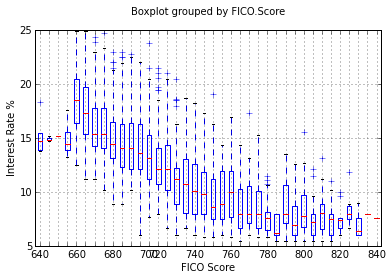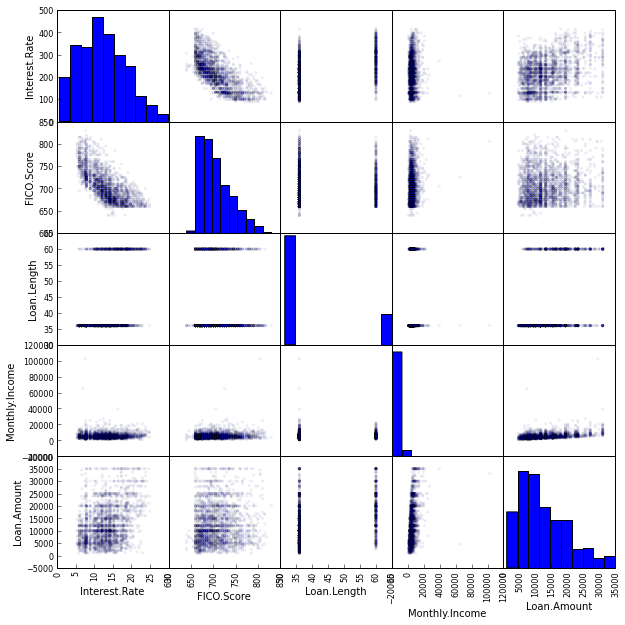## II. Data Cleanup¶

We find the data are "messy" i.e aren't cleanly prepared for import - for instance numeric columns might have some strings in them. This is very common in raw data especially that obtained from web sites.

Let's take a look. we're going to look at the first five rows of some specific columns that show the data dirtiness issues.

In :
%pylab inline
# first we ingest the data from the source on the web
# this contains a reduced version of the data set from Lending Club
import pandas as pd
loansData = pd.read_csv('https://spark-public.s3.amazonaws.com/dataanalysis/loansData.csv')

Populating the interactive namespace from numpy and matplotlib

In :
loansData['Interest.Rate'][0:5] # first five rows of Interest.Rate

Out:
81174     8.90%
99592    12.12%
80059    21.98%
15825     9.99%
33182    11.71%
Name: Interest.Rate, dtype: object
In :
loansData['Loan.Length'][0:5] # first five rows of Loan.Length

Out:
81174    36 months
99592    36 months
80059    60 months
15825    36 months
33182    36 months
Name: Loan.Length, dtype: object

We see here that:

• the interest rate information has "%" symbols in it.
• loan length has " months" in it

Other than that we can also see (exploration exercise):

• there are a couple of values that are so large they must be typos
• some values are missing "NA" values i.e. not available.
• the FICO Range is really a numeric entity but is represented as a categorical variable in the data.
In :
loansData['FICO.Range'][0:5] # first five rows of FICO.Range

Out:
81174    735-739
99592    715-719
80059    690-694
15825    695-699
33182    695-699
Name: FICO.Range, dtype: object

## Exercise¶

Actually perform each of the above steps on the dataset i.e.

• import the data
• remove the '%' suffix from each row
• remove the ' months' suffix from each row
• remove the outlier rows
• remove rows with NA

Save your code in a reusable manner - these are steps you'll be doing repeatedly.

In :
import matplotlib.pyplot as plt
import pandas as pd
plt.figure()
loansmin = pd.read_csv('../datasets/loanf.csv')
fico = loansmin['FICO.Score']
p = fico.hist()### Box Plot¶

Next we take a box plot which allows us to quickly look at the distribution of interest rates based on each FICO score range.

In :
import matplotlib.pyplot as plt
import pandas as pd
plt.figure()
loansmin = pd.read_csv('../datasets/loanf.csv')

p = loansmin.boxplot('Interest.Rate','FICO.Score')
q = p.set_xticklabels(['640','','','','660','','','','680','','','','700',
'720','','','','740','','','','760','','','','780','','','','800','','','','820','','','','840'])

q0 = p.set_xlabel('FICO Score')
q1 = p.set_ylabel('Interest Rate %')
q2 = p.set_title('                          ')We're going to look at a scatterplot matrix of the five variables in our data.In :
## TRY THIS!
import pandas as pd
loansmin = pd.read_csv('../datasets/loanf.csv')
a = pd.scatter_matrix(loansmin,alpha=0.05,figsize=(10,10), diagonal='hist')
## Click on the line above
## Change 'hist' to 'kde' then hit shift-enter, with the cursor still in this box
## The plot will redraw - it takes a while. While it is recomputing you will see a
## message-box that says 'Kernel Busy' near the top right corner
## You can change the code and hit shift-enter to re-execute the code
## Try changing the (10,10) to (8,8) and (12,12)
## Try changing the alpha value from 0.05 to 0.5
## How does this change in alpha change your ability to interpret the data?
## Feel free to try other variations.
## If at any time you scramble the code and forget the syntax
## a copy of the original code is below. Copy and paste it in place.
## Remember to remove the hashmarks.
## a = pd.scatter_matrix(loansmin, alpha=0.05,figsize=(10,10), diagonal='hist)In :
from IPython.core.display import HTML
def css_styling():
styles = open("../styles/custom.css", "r").read()
return HTML(styles)
css_styling()

Out: